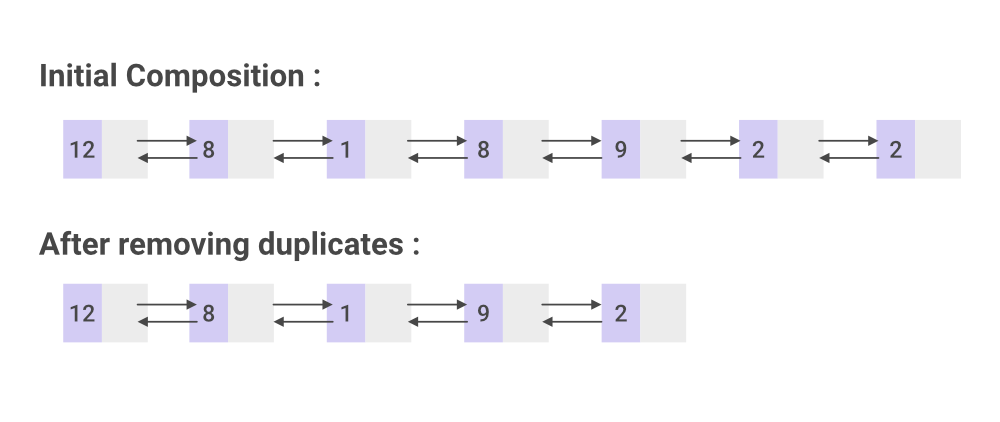# How to Remove Duplicate Elements From Java LinkedList?

• Difficulty Level : Easy
• Last Updated : 14 May, 2021

Linked List is a part of the Collection in java.util package. LinkedList class is an implementation of the LinkedList data structure it is a linear data structure. In LinkedList due to the dynamical allocation of memory, insertions and deletions are easy processes. For removing duplicates from

Example:

Attention reader! Don’t stop learning now. Get hold of all the important Java Foundation and Collections concepts with the Fundamentals of Java and Java Collections Course at a student-friendly price and become industry ready. To complete your preparation from learning a language to DS Algo and many more,  please refer Complete Interview Preparation Course.

```Initial composition :
7 2 3 3 2 7 6 2
After removing duplicates :
7 2 3 6```

Pictorial Representation : ( a node in a LinkedList has two parts : data and link to next node (null in case of the last element)#### Algorithm :

1. Initially, a  new node is created which points to the head.
2. A temp node will point to current and index node will point to current.next.
3. If the data of the index node and the current node is same i.e if a duplicate element is found, temp.next is made to point to index.next i.e it skips the duplicate element.
4. If the above condition is not satisfied, then the temp is made to point to the previous node of an index.
5. Index node iterates until the end and steps 3 and 4 are repeated.
6. Steps 2 to 5 are executed till the current node points to the end i.e reaches its end.

Below is the implementation of the above approach:

## Java

 `// Java Program to Remove Duplicate Elements From LinkedList``import` `java.io.*;` `// Creating the node class for a singly linkedlist``class` `Node {``    ``Node next;``    ``int` `data;` `    ``public` `Node(``int` `data)``    ``{``        ``this``.data = data;``        ``this``.next = ``null``;``    ``}``}` `public` `class` `singlyLinkedList {` `    ``// Defining the head and tail of a singly linkedlist``    ``public` `Node head = ``null``;``    ``public` `Node tail = ``null``;` `    ``// creating add() that enables addition``    ``// of a new node to the list``    ``public` `void` `add(``int` `data)``    ``{``        ``// Creating a new node``        ``Node newNode = ``new` `Node(data);` `        ``// Checking whether the list is empty or not``        ``if` `(head == ``null``) {``            ``// If the list is found to be empty, both head``            ``// and tail are made to point to  the new node``            ``head = newNode;``            ``tail = newNode;``        ``}``        ``else` `{``            ``// newNode is added after tail in such a way``            ``// that next node of the tail points to newNode``            ``tail.next = newNode;``            ``// newNode becomes the new tail of the list``            ``tail = newNode;``        ``}``    ``}` `    ``// Creating removeDuplicates() to remove``    ``// duplicates from the linkedlist``    ``public` `void` `removeDuplicates()``    ``{``        ``// current node points to the head element``        ``Node current = head, index = ``null``, temp = ``null``;` `        ``if` `(head == ``null``) {``            ``return``;``        ``}``        ``else` `{``            ``while` `(current != ``null``) {``                ``// temp node points to the previous node``                ``temp = current;``                ``// index node points to node next to current``                ``index = current.next;` `                ``while` `(index != ``null``) {``                    ``// checking if node of current data is``                    ``// equal to index node data``                    ``if` `(current.data == index.data) {` `                        ``// duplicate node is skipped``                        ``temp.next = index.next;``                    ``}``                    ``else` `{``                        ``// temp node points to the previous``                        ``// node of index node``                        ``temp = index;``                    ``}``                    ``index = index.next;``                ``}``                ``current = current.next;``            ``}``        ``}``    ``}` `    ``// creating print() to print all the data``    ``// of nodes present in the list``    ``public` `void` `print()``    ``{``        ``// Node current will point to head``        ``Node current = head;``        ``if` `(head == ``null``) {``            ``System.out.println(``                ``"Empty list please insert some elements first"``);``            ``return``;``        ``}``        ``while` `(current != ``null``) {` `            ``System.out.print(current.data + ``" "``);``            ``// incrementing pointer``            ``current = current.next;``        ``}``        ``System.out.println();``    ``}` `    ``public` `static` `void` `main(String[] args)``    ``{` `        ``singlyLinkedList List = ``new` `singlyLinkedList();` `        ``// Adding data to the list``        ``List.add(``9``);``        ``List.add(``1``);``        ``List.add(``1``);``        ``List.add(``3``);``        ``List.add(``4``);``        ``List.add(``8``);``        ``List.add(``2``);``        ``List.add(``1``);` `        ``System.out.println(``"Initial composition : "``);``        ``List.print();` `        ``// removing duplicate nodes``        ``List.removeDuplicates();` `        ``System.out.println(``"After removing duplicates : "``);``        ``List.print();``    ``}``}`
Output
```Initial composition :
9 1 1 3 4 8 2 1
After removing duplicates :
9 1 3 4 8 2
```

Time Complexity: O(N2)

My Personal Notes arrow_drop_up